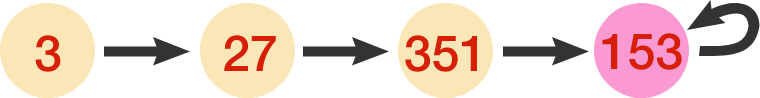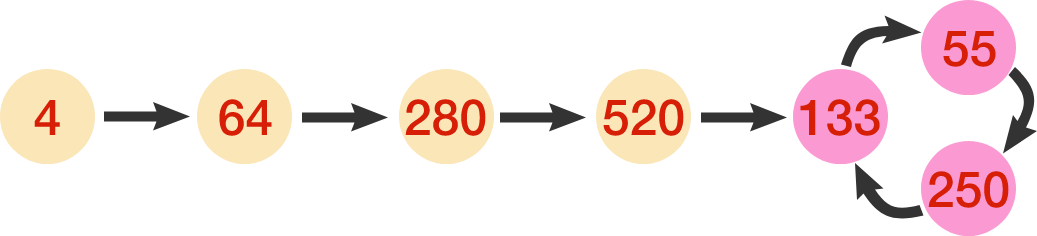# Digits Cubes Limit

$f(n)$ gives the sum of the cubed digits of some positive integer $n.$ For example, $f(123)=1^3+2^3+3^3=36.$

If we repeatedly apply this process on each previous result, the following two different behaviors may arise:

• Arrive at a fixed point: For example, beginning with 3, we eventually arrive at 153, and $f(153)=153.$ We say that 153 is a fixed point.• Arrive at a limit cycle: For example, beginning with 4, we eventually arrive at 133, and $f(133) = 55 \implies f(55) = 250 \implies f(250)=133.$ We say that $\{55,133,250\}$ is a limit cycle.Let the limit set be the set of all fixed points and limit cycles in the range of $f(n).$

Find the sum of all the numbers in the limit set (including the four in pink found above). Note: A coding environment is provided below:

You need to be connected to run code

Bonus: Prove that the limit set actually contains finitely many numbers.

×